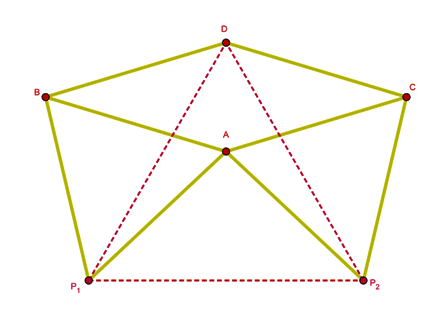All constructions that can be made using straight edge and compass can be made using matchsticks (identical moveable line segments).

(This can also be considered as rusty compass constructions in which the compass is fixed and does not match any given length).

Here is the construction of an equilateral triangle formed on the base $P_1P_2$Construct each point in alphabetical order.

Prove that the red dotted triangle $DP_1P_2$ is equilateral for all bases $P_1P_2$ < two match length